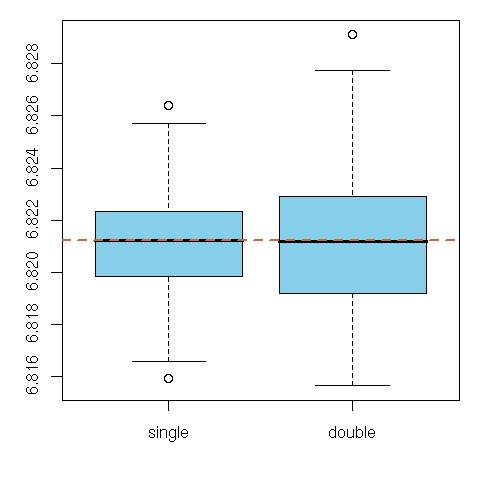# Multidimension bridge sampling (CoRe in CiRM )

July 13, 2010
By

(This article was first published on Xi'an's Og » R, and kindly contributed to R-bloggers)Since Bayes factor approximation is one of my areas of interest, I was intrigued by Xiao-Li Meng’s comments during my poster in Benidorm that I was using the “wrong” bridge sampling estimator when trying to bridge two models of different dimensions, based on the completion (for$theta_2=(mu,sigma^2)$ and$mu=theta_1$ missing from the first model)$B^pi_{12}(x)= dfrac{displaystyle{intpi_1^*(mu|sigma^2){tildepi}_1(sigma^2|x) alpha(theta_2) {pi}_2(theta_2|x)hbox{d}theta_2}}{ displaystyle{int{tildepi}_2(theta_2|x)alpha(theta_2) pi_1(sigma^2|x)hbox{d}sigma^2 } pi_1^*(mu|sigma^2) hbox{d}mu },.$

When revising the normal chapter of Bayesian Core,  here in CiRM, I thus went back to Xiao-Li’s papers on the topic to try to fathom what the “true” bridge sampling was in that case. In Meng and Schilling (2002, JASA), I found the following indication, “when estimating the ratio of normalizing constants with different dimensions, a good strategy is to bridge each density with a good approximation of itself and then apply bridge sampling to estimate each normalizing constant separately. This is typically more effective than to artificially bridge the two original densities by augmenting the dimension of the lower one”. I was unsure of the technique this (somehow vague) indication pointed at until I understood that it meant  introducing one artificial posterior distribution for each of the parameter spaces and processing each marginal likelihood as an integral ratio in itself. For instance, if$eta_1(theta_1)$ is an arbitrary normalised density on$theta_1$, and$alpha$ is an arbitrary function, we have the bridge sampling identity on$m_1(x)$:$inttilde{pi}_1(theta_1|x) ,text{d}theta_1 = dfrac{displaystyle{int tilde{pi}_1(theta_1|x) alpha(theta_1) {eta}_1(theta_1),text{d}theta_1}}{displaystyle{inteta_1(theta_1) alpha(theta_1) pi_1(theta_1|x) ,text{d}theta_1}}$

Therefore, the optimal choice of$alpha$ leads to the approximation$widehat m_1(x) = dfrac{displaystyle{sum_{i=1}^N {tildepi}_1(theta^eta_{1i}|x)big/left{{m_1(x) tildepi}_1(theta^eta_{1i}|x) + eta(theta^eta_{1i})right}}}{displaystyle{ sum_{i=1}^{N} eta(theta_{1i}) big/ left{{m_1(x) tildepi}_1(theta_{1i}|x) + eta(theta_{1i})right}}}$

when$theta_{1i}simpi_1(theta_1|x)$ and$theta^eta_{1i}simeta(theta_1)$. More exactly, this approximation is replaced with an iterative version since it depends on the unknown$m_1(x)$. The choice of the density$eta$ is obviously fundamental and it should be close to the true posterior$pi_1(theta_1|x)$ to guarantee good convergence approximation. Using a normal approximation to the posterior distribution of$theta$ or a non-parametric approximation based on a sample from$pi_1(theta_1|mathbf{x})$, or yet again an average of MCMC proposals are reasonable choices.The boxplot above compares this solution of Meng and Schilling (2002, JASA), called double (because two pseudo-posteriors$eta_1(theta_1)$ and$eta_2(theta_2)$ have to be introduced), with Chen, Shao and Ibragim (2001) solution based on a single completion$pi_1^*$ (using a normal centred at the estimate of the missing parameter, and with variance the estimate from the simulation), when testing whether or not the mean of a normal model with unknown variance is zero. The variabilities are quite comparable in this admittedly overly simple case. Overall, the performances of both extensions are obviously highly dependent on the choice of the completion factors,$eta_1$ and$eta_2$ on the one hand and$pi_1^*$ on the other hand, . The performances of the first solution, which bridges both models via$pi_1^*$, are bound to deteriorate as the dimension gap between those models increases. The impact of the dimension of the models is less keenly felt for the other solution, as the approximation remains local.

R-bloggers.com offers daily e-mail updates about R news and tutorials on topics such as: Data science, Big Data, R jobs, visualization (ggplot2, Boxplots, maps, animation), programming (RStudio, Sweave, LaTeX, SQL, Eclipse, git, hadoop, Web Scraping) statistics (regression, PCA, time series, trading) and more...

Tags: , , , , , , , , ,